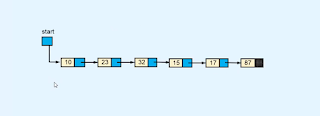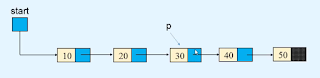# Traversing in Linked list | Data structures

we can traverse in a linked list using the links of every node. as we know the single linked list is made up of a node where each node has two parts. one is the info part and the other one link part.### Info part of the list

The info part contains the actual data to be stored on the list.

### Linked part of the list

link is a reference to the next value of the list.in a single linked list, we maintain a reference point to the first value of the linked list and we named it to start variable.

it's the identity of the list and with the only help of the start variable, we can access the whole list following the links of each node.

if a node contains no value or None. this means the linked list ended up there. in the above image. the linked part of the node that contains the value None is the last node of the list.

and for an empty linked list, the start variable contains the value None.

## Traversing into list

Traversing means we can visit each node in a list at a single time.

to traverse into a list we need to know how we can move forward using the link of the list. suppose we have a reference to the node as you see in the image.so we write

` p = p.link`

so this refers to the next node of the list as you see in the image.Now to traverse into the linked list we need to refer to the first node of the list as you see in the image.so we point the variable p to the first node of the list using the code.

`  self.start = None`

now using this code we can traverse the whole list.

```def display_list(self):
if self.start is None:
print("List is empty")
return    else:
print("List is :   ")
p = self.start
while p is not None:
print(p.info, " ", end=' ')
print()```

#### Python program for traversing in the linked list

```class Node:

def __init__(self, value):
self.info = value

def __init__(self):
self.start = None
def display_list(self):
if self.start is None:
print("List is empty")
return        else:
print("List is :   ")
p = self.start
while p is not None:
print(p.info, " ", end=' ')
print()

def count_nodes(self):
p = self.start
n = 0        while p is not None:
n += 1            p = p.link
print("Number of nodes in the list = ", n)

def search(self):
position = 1        p = self.start
while p is not None:
if p.info == x:
print(x, " is at position ", position)
return True            position += 1            p = p.link
else:
return False
def insert_in_beginning(self, data):
temp = Node(data)
self.start = temp

def insert_at_end(self, data):
temp = Node(data)
if self.start is None:
self.start = temp
return
p = self.start

def create_list(self):
n = int(input("Enter the number of nodes  :  "))
if n == 0:
return        for i in range(n):
data = int(input("Enter the element to be inserted : "))
self.insert_at_end(data)

def insert_after(self, data, x):
p = self.start
while p is not None:
if p.info == x:

if p is None:
print(x, "not present in the list")
else:
temp = Node(data)

def insert_before(self, data, x):
# If list is empty        if self.start is None:
print("List is empty")
return
if x == self.start.info:
temp = Node(data)
self.start = temp
return

p = self.start

print(x, " not present in the list")
else:
temp = Node(data)

def insert_at_position(self, data, k):
if k == 1:
temp = Node(data)
self.start = temp
return
p = self.start
i = 1        while i < k - 1 and p is not None:
i += 1
if p is None:
print("You can insert only upto position", i)
else:
temp = Node(data)

def delete_node(self, x):

if self.start is None:
print("List is empty")

if self.start.info == x:
return
p = self.start

print("Element ", x , "not in list")
else:

def delete_first_node(self):
if self.start is None:

def delete_last_node(self):

if self.start is None:
return
self.start = None            return
p = self.start
def reverse_list(self):

prev = None        p = self.start
while p is not None:
prev = p
p = next
self.start = prev

def bubble_sort_exdata(self):

end = None
p = self.start
if p.info > q.info:
p.info, q.info = q.info, p.info
end = p

end = None
r = p = self.start
if p.info > q.info :
if p != self.start:
else:
self.start = q
p,q = q,p
r = p
end = p

def has_cycle(self):
if self.find_cycle() is None:
return False        else:
return True
def find_cycle(self):
if self.start is None or self.start.link is None:
return None
slowR = self.start
fastR = self.start

while fastR is not None and fastR.link is not None:
if slowR == fastR:
return slowR
return None
def remove_cycle(self):
c = self.find_cycle()
if c is None:
return        print("Node at which the cycle was detected is ", c.info)

p = c
q = c
len_cycle = 0
while True:
if p == q:
break        print("Length of cycle is :", len_cycle)

len_rem_list = 0        p = self.start
while p != q:

print("Number of nodes not included in the cycle are : ", len_rem_list)
length_list = len_cycle + len_rem_list
print("Length of the list is : ", length_list)

p = self.start
for i in range(length_list-1):

def insert_cycle(self, x):
if self.start is None:
return        p = self.start
px = None        prev = None
while p is not None:
if p.info == x:
px = p
prev = p

if px is not None:
else:
print(x, " not present in list")

def merge2(self, list2):
merge_list.start = self._merge2(self.start, list2.start)
return merge_list

def _merge2(self, p1, p2):
if p1.info <= p2.info:
startM = p1
else:
startM = p2
pM = startM

while p1 is not None and p2 is not None:
if p1.info <= p2.info:
else:

if p1 is None:
else:

return startM

def merge_sort(self):
self.start = self._merge_sort_rec(self.start)

def _merge_sort_rec(self, list_start):

if list_start is None or list_start.link is None:
return list_start

start1 = list_start
start2 = self._divide_list(list_start)
start1 = self._merge_sort_rec(start1)
start2 = self._merge_sort_rec(start2)
startM = self._merge2(start1, start2)

return startM

def _divide_list(self, p):
while q is not None and q.link is not None:

##########################

list.create_list()

while True:
print("1. Display list")
print("2. Count the number of nodes")
print("3. Search for an element")
print("4. Insert in empty list/insert in beginning of the list")
print("5. Insert a node at the end of the list")
print("6. Insert a node after a specified node")
print("7. Insert a node before a specified node")
print("8. Insert a node at a given position")
print("9. Delete first node")
print("10. Delete last node")
print("11. Delete any node")
print("12. Reverse the list")
print("13. Bubble sort by exchanging data")
print("14. Bubble sort by exchanging links")
print("15. Merge sort")
print("16. Insert Cycle")
print("17. Detect Cycle")
print("18. Remove Cycle")
print("19. Quite")

if option == 1:
list.display_list()
elif option == 2:
list.count_nodes()
elif option == 3:
data = int(input("Enter the element to be searched : "))
list.search(data)
elif option == 4:
data = int(input("Enter the element to be inserted : "))
list.insert_in_beginning(data)
elif option == 5:
data = int(input("Enter the element to be inserted : "))
list.insert_at_end(data)
elif option == 6:
data = int(input("Enter the element to be inserted : "))
x = int(input("Enter the element after which to insert : "))
list.insert_after(data, x)
elif option == 7:
data = int(input("Enter the element to be inserted : "))
x = int(input("Enter the element before which to insert : "))
list.insert_before(data, x)
elif option == 8:
data = int(input("Enter the element to be inserted : "))
k = int(input("Enter the position at which to insert : "))
list.insert_at_position(data, k)
elif option == 9:
list.delete_first_node()
elif option == 10:
list.delete_last_node()
elif option == 11:
data = int(input("Enter the element to be deleted : "))
list.delete_node(data)
elif option == 12:
list.reverse_list()
elif option == 13:
list.bubble_sort_exdata()
elif option == 14:
elif option == 15:
list.merge_sort()
elif option == 16:
data = int(input("Enter the element at which the cycle has to be inserted : "))
list.insert_cycle(data)
elif option == 17:
if list.has_cycle():
print("List has a cycle")
else:
print("List does not have a cycle")
elif option == 18:
list.remove_cycle()
elif option == 19:
break    else:
print("Wrong option")
print()```